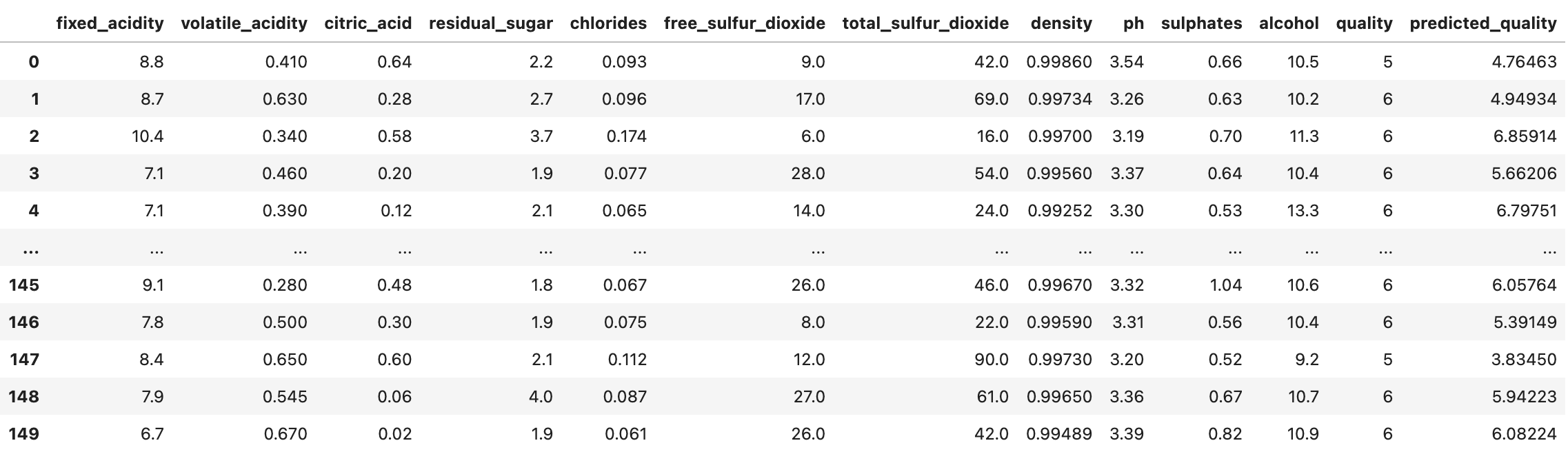# Registering a Regression Model

Suppose you would like to register a regression model for the following dataset.Following is an example of how you would construct a `fdl.ModelInfo` object and register such a model.

``````PROJECT_ID = 'example_project'
DATASET_ID = 'wine_data'
MODEL_ID = 'regression_model'

dataset_info = client.get_dataset_info(
project_id=PROJECT_ID,
dataset_id=DATASET_ID
)

model_target = 'quality'
model_outputs = ['predicted_quality']
model_features = [
'fixed_acidity',
'volatile_acidity',
'citric_acid',
'residual_sugar',
'chlorides',
'free_sulfur_dioxide',
'total_sulfur_dioxide',
'density',
'ph',
'sulphates',
'alcohol'
]

model_info = fdl.ModelInfo.from_dataset_info(
dataset_info=dataset_info,
dataset_id=DATASET_ID,
target=model_target,
outputs=model_outputs,
)

client.register_model(
project_id=PROJECT_ID,
dataset_id=DATASET_ID,
model_id=MODEL_ID,
model_info=model_info
)
``````

### 🚧Note

If you do not provide model predictions in the DataFrame used to infer the `fdl.DatasetInfo` object, you’ll need to pass a dictionary into the `outputs` argument of `fdl.ModelInfo.from_dataset_info` that contains the min and max values for the model output.

``````model_outputs = {
'predicted_quality': (0.0, 1.0)
}
``````